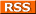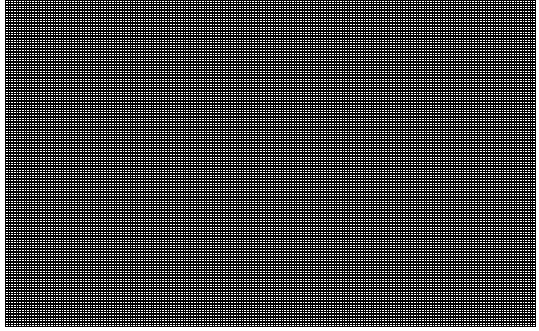Comments Rss
PostGIS: ST_Translate

# ST_Translate, Translate

The ST_Translate function takes any geometry (linestring, multiline etc) returns a new geometry that is the original geometry moved by a vector defined by X,Y,Z. Note the units of measurement are always in the units of the spatial reference system of the geometry argument. There are two forms of it ST_Translate. ST_Translate(geometry, X, Y, Z) and ST_Translate(geometry, X,Y).

In the below example we use the translate function to create a grid that divides the extent of Boston into into 200x200 rectangles (40,000). By doing the following

• Create a reference box starting at the origin of our extent of Massachusetts that is of dimension 1485x911 meters - in quasi OGC notation - BOX(xorigin yorigin, (xorigin + 1485) (yorigin + 911)) -> BOX(33863.73046875 777606.3125,35348.73046875 778517.3125)
• Next take this box and use it as a paint brush to paint across and then down by translating it hor.n*1485, ver.n*911
``` CREATE TABLE throwaway_grid(gid SERIAL PRIMARY KEY); SELECT AddGeometryColumn('public', 'throwaway_grid', 'the_geom', 26986, 'POLYGON', 2); INSERT INTO throwaway_grid(the_geom) SELECT ST_translate(ref.boxrep, hor.n*width, ver.n*height) As slice FROM (SELECT ST_xmin(ST_Extent(the_geom)) As xstart, ST_xmin(ST_Extent(the_geom)) as ystart, ST_SetSRID(CAST('BOX(33863.73046875 777606.3125,35348.73046875 778517.3125)' as box2d), 26986) as boxrep, ceiling((ST_xmax(ST_Extent(the_geom)) - ST_xmin(ST_Extent(the_geom)))/200) as width, ceiling((ST_ymax(ST_Extent(the_geom)) - ST_ymin(ST_Extent(the_geom)))/200) as height FROM towns) As ref, generate_series(1,200) as hor(n), generate_series(1,200) as ver(n); CREATE INDEX idx_throwaway_grid_the_geom ON throwaway_grid USING gist(the_geom); ```

The resulting image of this looks like this.Take a look at Map Dicing to see an example of how you can use this in conjunction with ST_Interseciton to dice up maps into smaller pieces.

The translate function can be used in conjunction with other functions such as Rotate to do interesting things like rotate a geometry about a point or create ellipses such as described here